# 卡尔曼滤波实战 | 形象化理解卡尔曼滤（附源码） 原创 精华

4收藏

## 一、概述

PS：这篇文章有大部分来自外国的一篇课程讲义：Understanding the Basis of the Kalman Filter Via a Simple and Intuitive Derivation。当然，语言是自己重新组织，同时，符号上的规定保持与本专栏卡尔曼滤波系列的其他文章一致。

## 二、最小例子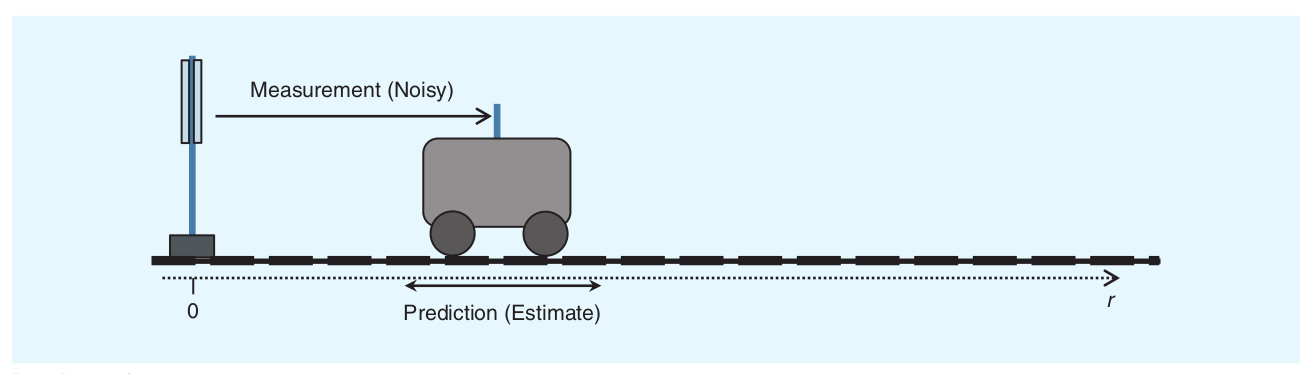## 三、卡尔曼滤波的两个步骤

1. 预测（prediction）—— Dynamic model
2. 更新（correction/measurment update）—— Observation model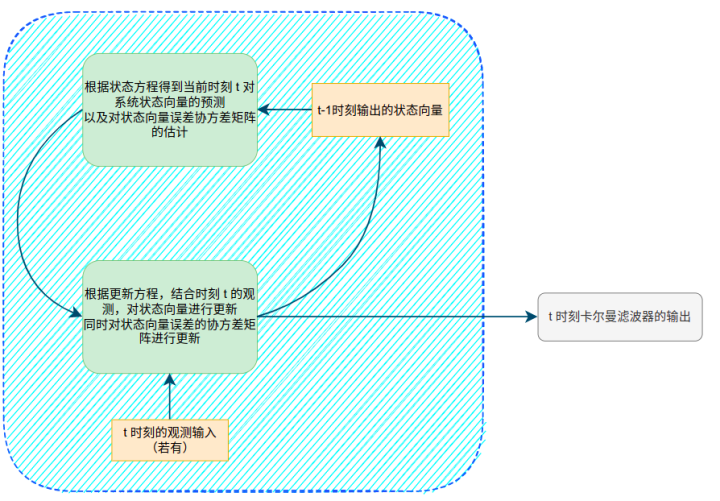## 四、卡尔曼滤波的状态方程

### 状态方程

$$\textbf{x}_t = \textbf{A}t\textbf{x}{t-1}+ \textbf{B}_t\textbf{u}_t+\textbf{w}_t \tag{1}$$

• $\textbf{x}_t$就是每一时刻系统的状态向量，N行1列
• $\textbf{u}_t$是该时刻系统的输入，M行1列
• $\textbf{w}_t$是系统随机噪声，N行1列；且该随机噪声是高斯噪声，均值为0，协方差矩阵为$\textbf{Q}_t$，N行N列
• $\textbf{A}_t$叫做状态转换矩阵，N行N列
• $\textbf{B}_t$称为控制矩阵，N行M列

PS:大写字母表示矩阵；小写加粗字母表示向量；小写字母表示变量

$$\hat{\textbf{x}}^-_t = \textbf{A}t\hat{\textbf{x}}^+{t-1} + \textbf{B}_t\textbf{u}_t\tag{2}$$

• 上标$\hat{.}$表示该值是一个估计的量，角标+、-表示更新后，更新前的量。

（当前时刻预测的状态向量经过第二步骤的更新之后成为当前时刻的最终输出）

### 代入例子中

$$\textbf{x}t = \begin{bmatrix} d_t\ v_t \end{bmatrix}$$

$$\textbf{u}t=[a_t]$$

$$v_t=v {t-1}+a_t\Delta t$$
$$d_t = d {t-1}+v {t-1}\Delta t+\frac{1}{2}a_t\Delta t^2$$

$$\textbf{x}t = \begin{bmatrix} d_t\ v_t \end{bmatrix}= \begin{bmatrix} 1 \ \ \Delta t\ 0 \ \ \ \ \ 1\ \end{bmatrix} \begin{bmatrix} d{t-1}\ v {t-1}\ \end{bmatrix}+ \begin{bmatrix} \frac{\Delta t^2}{2}\ \Delta t\ \end{bmatrix}a_t\$$

$$\textbf{A}_t= \begin{bmatrix} 1 \ \ \Delta t\ 0 \ \ \ \ \ 1\ \end{bmatrix}, \textbf{B}_t= \begin{bmatrix} \frac{\Delta t^2}{2}\ \Delta t\ \end{bmatrix} \$$

## 五、状态向量的协方差矩阵

### 协方差矩阵的推导

$$\begin{split}P^-t&=\mathbb{E}[\big((A_tx{t-1}+w_t)-A_tx_{t-1}^+\big)\big((A_tx_{t-1}+w_t)-A_tx_{t-1}^+\big)^T]\ &=\mathbb{E}[\big(A_t(x_{t-1}-x_{t-1}^+)+w_t\big)\big(A_t(x_{t-1}-x_{t-1}^+)+w_t\big)^T]\ &=\mathbb{E}[A_t(x_{t-1}-x_{t-1}^+)(x_{t-1}-x_{t-1}^+)^TA_t^T]+\mathbb{E}[w_tw_t^T]\ &=A_tP_{t-1}^+A_t^T+Q_t \end{split} \$$

$$P^-t=A_tP^+{t-1}A^T_t+Q_t\tag{3}\$$

### 代入例子中

$$\textbf{P}_0= \begin{bmatrix}10^{-5} \ \ 0\0 \ \ 10^{-5}\ \end{bmatrix}, \textbf{Q}_0= \begin{bmatrix}10^{-10} \ \ 0\ 0 \ \ \ 10^{-10}\ \end{bmatrix}$$

## 六、如何理解预测方程和协方差矩阵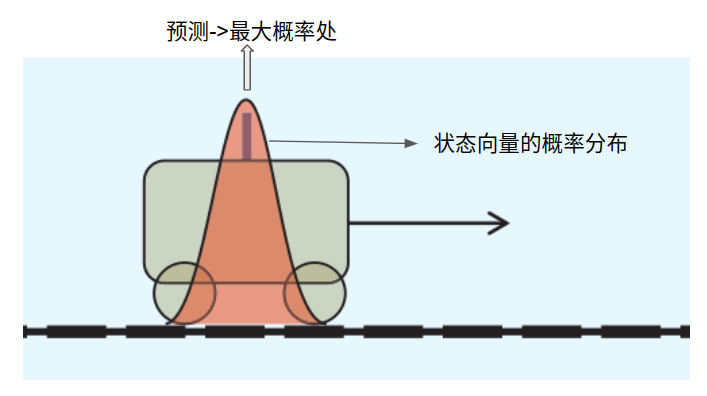$$\textbf{x}_t = \textbf{A}t\textbf{x}{t-1}+ \textbf{B}_t\textbf{u}_t+\textbf{w}_t$$

$$\hat{\textbf{x}}^-_t = \textbf{A}t\hat{\textbf{x}}^+{t-1} + \textbf{B}_t\textbf{u}_t$$

## 七、测量更新

### 测量

$$\textbf{z}_t=H_t\textbf{x}_t+\textbf{v}_t\tag{4}\$$

• $\textbf{z}_t$是测量向量，L行1列
• $H_t$就叫做测量转换矩阵吧（不知道可不可以这样叫），L行N列。作用就是将系统状态映射到测量域
• $\textbf{v}_t$叫做测量噪声，L行1列。其协方差矩阵为$\textbf{R}_t$
• 我们同时假设$\bar{\textbf{z}}_t$是测量得到的值，这个值也是$\textbf{z}_t$的均值

### 代入例子中

$$\textbf{x}_t = \begin{bmatrix} d_t\ v_t \end{bmatrix}$$

$$\begin{split} H_t= \begin{bmatrix} \frac{1}{c}\ \ 0 \end{bmatrix} \end{split}$$

### 测量更新

$$p(z)\sim N(\bar{z}, R)$$

$$\textbf{x}^2_t \sim N(H_t^{-T}\bar{\textbf{z}}_t, H_t^{-T}R_tH_t^{-1})$$

$$\textbf{x}^1_t \sim N(\hat{\textbf{x}}_t^-, P_t^-)$$

$$\begin{split} \mu_{fused}&=\frac{\mu_1\sigma_2^2+\mu_2\sigma_1^2}{\sigma_1^2+\sigma_2^2}\ &=\mu_1+\frac{(\mu_2-\mu_1)\sigma_1^2}{\sigma_1^2+\sigma_2^2}\ &=\hat{x}_t^-+\frac{(H_t^{-1}\bar{z}_t-\hat{x}_t^-)P_t^-}{P_t^-+H_t^{-1}R_t(H_t^{-1})^T}\ &=\hat{x}_t^-+\frac{P_t^-H_t^T}{H_tP_t^-H_t^T+R_t}(\bar{z}_t-H_t\hat{x}_t^-)\ &=\hat{x}_t^-+K_t(\bar{z}_t-H_t\hat{x}t^-) \end{split}\$$
$$\begin{split} \sigma {fused}^2 &=\frac{\sigma_1^2\sigma_2^2}{\sigma_1^2+\sigma_2^2}\ &=\sigma_1^2-\frac{\sigma_1^4}{\sigma_1^2+\sigma_2^2}\ &=P_t^–\frac{P_t^-H_t^TH_tP_t^-}{H_tP_t^-H_t^T+R_t}\ &=P_t^–K_tH_tP_t^- \end{split}\$$

$$K_t=\frac{P^-_tH_t^T}{H_tP^-_tH_t^T+R_t}$$

$$\hat{x}_t^+=\hat{x}_t^-+K_t(\bar{z}_t-H_t\hat{x}_t^-)$$

## 八、总结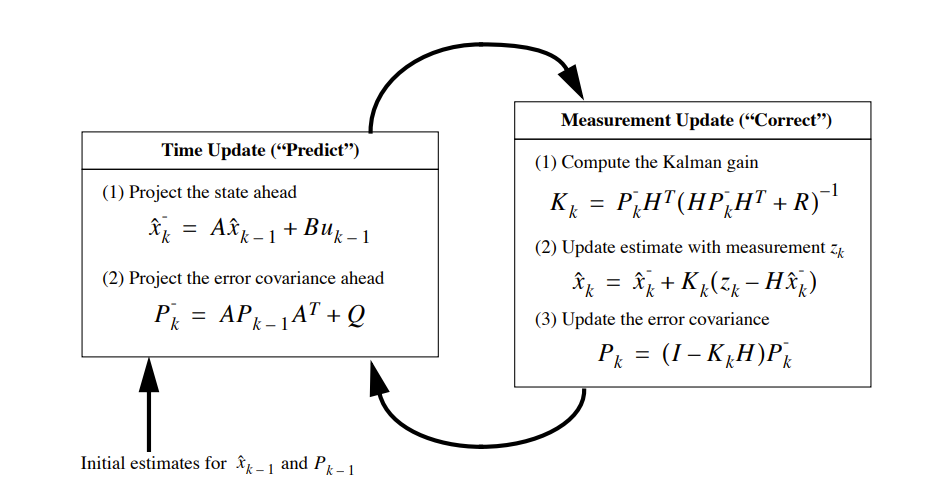## 九、代码

### 数据生成

import numpy as np
from matplotlib import pyplot as plt
C = 100  # velocity of light, just a simulation value
# generate perfect acceleration and corresponding velocity and distance
# 生成没有噪声的加速度信号，并用积分计算出对应的速度和距离 ---------------------------------
N_steps = 1000  # 1000 steps to estimate
Delta_t = 0.05  # time interval is 0.05s
timeline = np.arange(N_steps) * Delta_t  # \Delta t = 0.05s
accs_gt = np.sin(timeline)  # set accelerator as a log shape
vels_gt = np.cumsum(accs_gt*Delta_t)  # get the ground truth velocity;速度是加速度的积分
dists_gt = np.cumsum(vels_gt*Delta_t)  # get the ground truth distance; 距离是速度的积分
zs_gt = dists_gt/C
#----------------------------------

# add noise to acceleration signals and calculate the velocity and distance with simple integral
# 往加速度信号添加噪声模拟真实传感器信号，并用简单的积分计算速度和距离
accs_noise_var = 0.005  # set acceleration noise variation as 0.5
accs_noise = np.random.rand(N_steps) * accs_noise_var
accs_w_noise = accs_gt + accs_noise
vels_w_noise = np.cumsum(accs_w_noise*Delta_t)
# vels_w_noise = np.cumsum(accs_noise * Delta_t)
dists_w_noise = np.cumsum(vels_w_noise*Delta_t)
zs_noise_var = 0.001
zs_noise = np.random.rand(N_steps) * zs_noise_var
zs_w_noise = zs_gt + zs_noise
#---------------------------------


### 数据可视化结果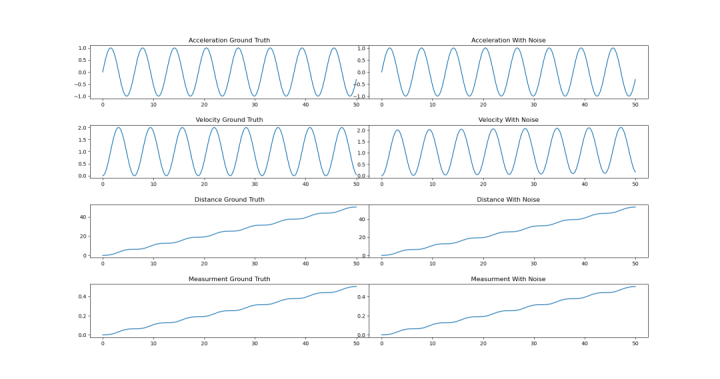### 各个转换矩阵及初始化

A = np.array([
[1, Delta_t],
[0, 1]
])  # 状态转移矩阵
B = np.array([
[Delta_t**2*0.5],
[Delta_t]
])  # 控制转移矩阵
Q_var = 0.005
Q = np.array([
[Q_var, 0],
[0, Q_var]
])  # 预测噪声协方差矩阵
P0 = np.array([
[0.0, 0],
[0.0, 0]
])  # 状态向量协方差初始值
H = np.array([
1/C, 0
]).reshape((1,2))  # 测量转换矩阵
R_var = 0.001
R = R_var  # 测量协方差矩阵，此时只有一个测量变量，因此只有一个值

x0 = np.array([
,

])  # 系统状态向量初始化，速度和距离均初始化为0


### 卡尔曼滤波过程

est_vel = 
est_dist = 
for i in range(N_steps-1):
if i == 0:
x_t = x0
P_t = P0
u = np.array([accs_w_noise[i-1]])
x_t_ = A@x_t + B*u  # 预测方程
P_t_ = A@P_t@(A.T) + Q  # 预测状态向量的协方差矩阵

K = P_t_@H.T / (H@P_t_@H.T + R)  # 卡尔曼增益
x_t = x_t_ + K*(zs_w_noise[i-1] - H@x_t_)  # 更新方程
P_t = P_t_ - K@H@P_t_  # 更新状态向量协方差矩阵

est_vel.append(x_t)
est_dist.append(x_t)


### 滤波结果可视化及误差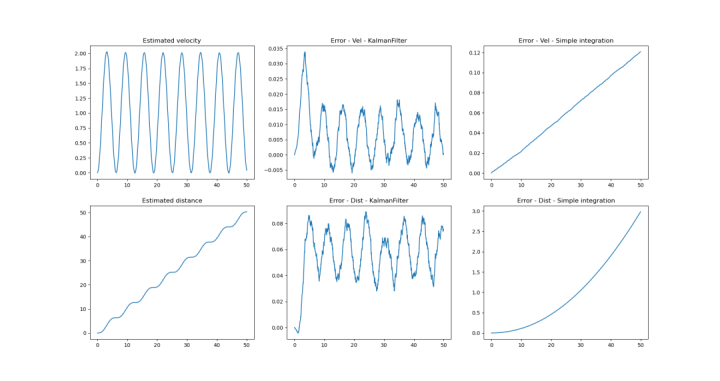## Ref

 Understanding the Basis of the Kalman Filter Via a Simple and Intuitive Derivation